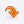UniversitiesLOGINCONTACTMost Recent ArticlesMost Rated ArticlesCategories

Travel/ TourismProject Topics
Project work for Discrete Mathematics
Author : shanu
 Category : Project Topics posted Date :
 Total No.of views : Total No.of Comments : 0
Rating:0 / 5 (0 votes)

Tags :
Project Topics for Discrete Mathematics, Algorithms, Graph Theory.

Project work for Discrete MathematicsDiscrete mathematics is the study of mathematical structures that are fundamentally discrete rather than continuous. In contrast to real numbers that have the property of varying "smoothly", the objects studied in discrete mathematics – such as integers, graphs, and statements in logic  do not vary smoothly in this way, but have distinct, separated values. Discrete mathematics therefore excludes topics in "continuous mathematics" such as calculus and analysis. Discrete objects can often be enumerated by integers. More formally, discrete mathematics has been characterized as the branch of mathematics dealing with countable set  (sets that have the same cardinality as subsets of the natural numbers, including rational numbers but not real numbers). Countable sets are sets that contain countable elements and can be indexed, such as 1, 2, 3, and so on. Discrete functions are functions that can only take in values from countable sets. For example, a discrete function cannot take in the number 3.14 as a value. Due to the breadth of topics in discrete math, it may be difficult narrowing down a topic for a discrete math project. However, you can consider topics in the major sub-fields of discrete math to decide on a more specific topic.

Combinatorics:
Combinatorics, in short, is the mathematics of counting. Questions in combinatorics include asking how many ways there are to complete a task or what number of possible outcomes there are for a specific event. For example, determining the total amount of beverage combinations for a group of people ordering from a set list of beverages is a discrete math problem. You can choose a topic in this area that looks at such theoretical questions as how many combinations exist for situations that involve choice, or you can focus on more applied questions, such as the number of options certain restaurants offer. For such topics, combinatorics would play the role of calculating the answers.

Boolean Algebra:
Boolean algebra is a relatively new area of discrete mathematics. This sub-field became noticed in the 1930s when it was used to analyze electric circuits. Using Boolean algebra, you can design circuits with specific functions in mind. For example, you can design a circuit that computes mathematical calculations, such as summing integers. Projects in this area may be as simple as designing circuits with specific uses or analyzing in terms of Boolean algebra how specific electronic devices work.

Algorithms:
You may have heard of algorithms as a type of computer programming tool. In fact, algorithms come from discrete math but are applied in many real-world situations. Algorithms are basically sets of rules that help to answer large sets of questions or prove mathematical theorems. Projects on algorithms should focus on answering a question through the application of algorithms. Examples of questions that algorithms can answer are "Does an integer between 0 and 1 exist?" and "Can any number greater than 2 be created simply through adding 2s and 3s?"

Graph Theory:
Graph theory, a sub-branch of discrete math, uses visual rendering of math problems to help mathematicians arrive at a solution. There are myriad applications for graph theory, and you can find a project topic easily by imagining a situation or by looking at pictures, such as maps or buildings. A couple of examples of projects in this area are how to connect utility lines to houses arranged in a certain order without having the lines cross, and how to move through a logistical route in the most efficient manner.